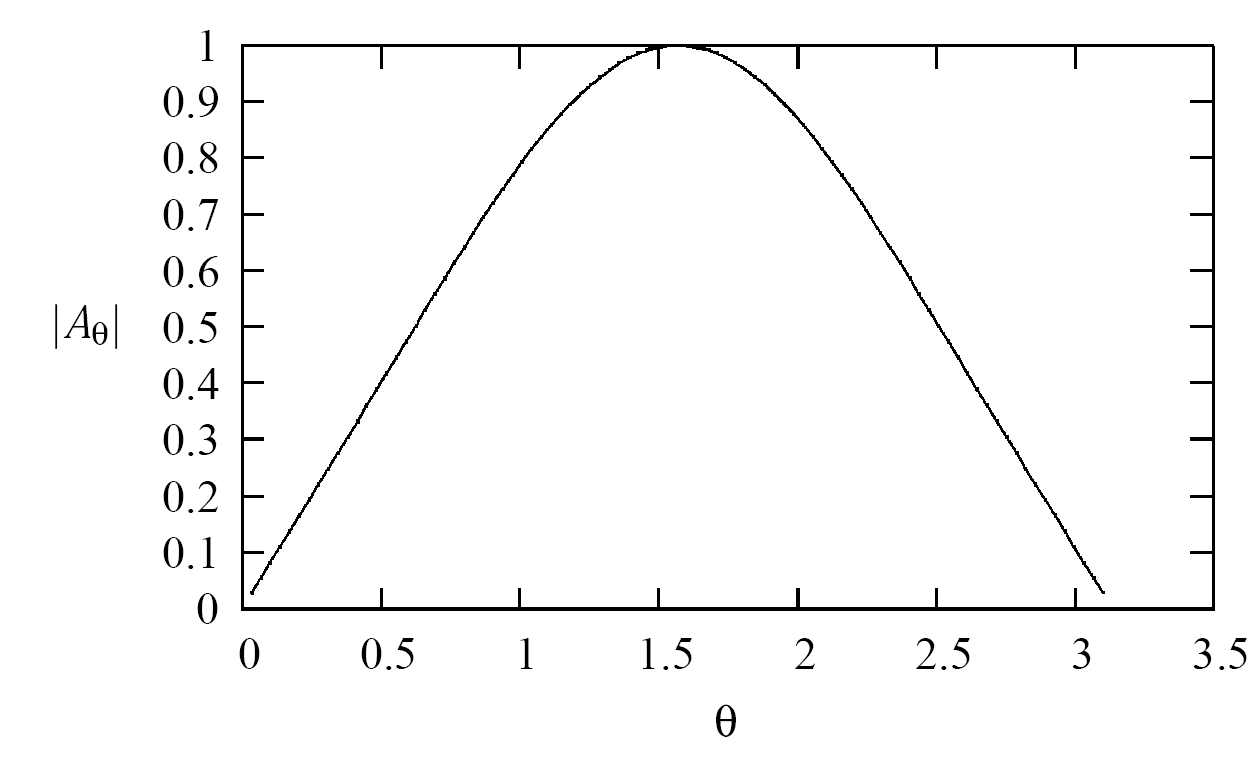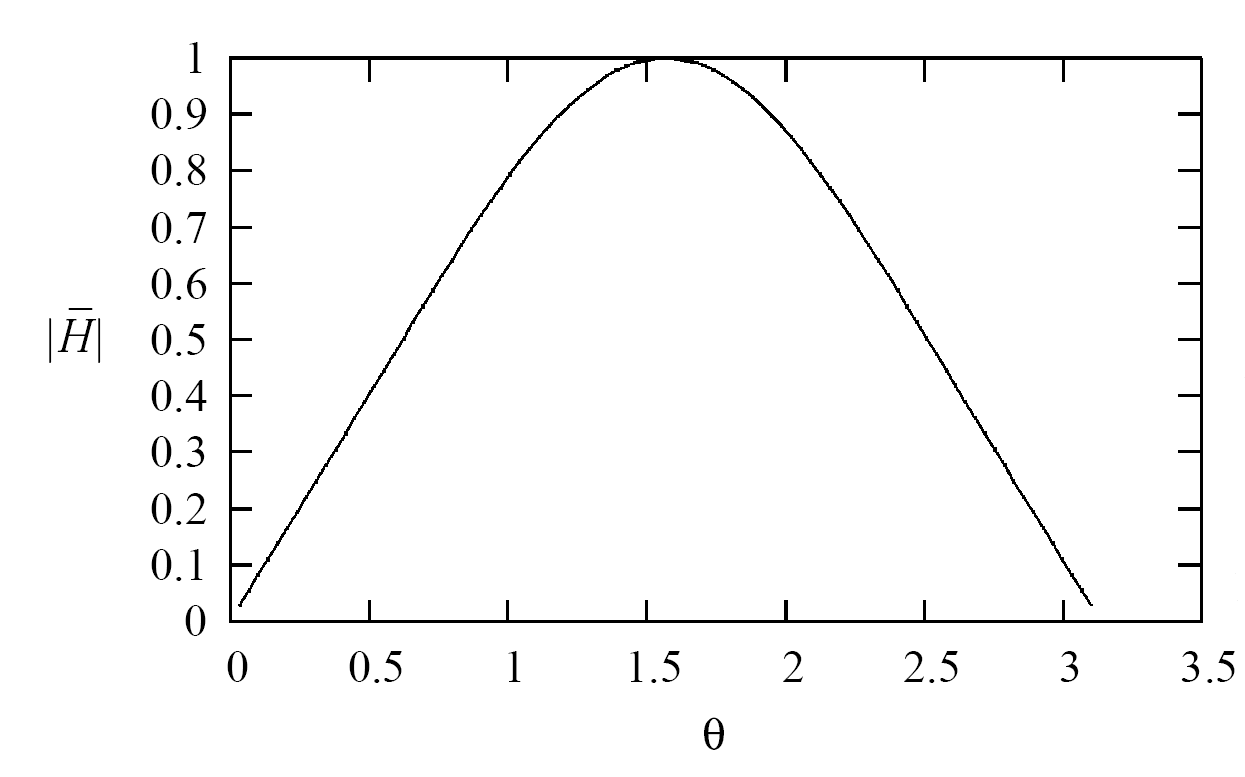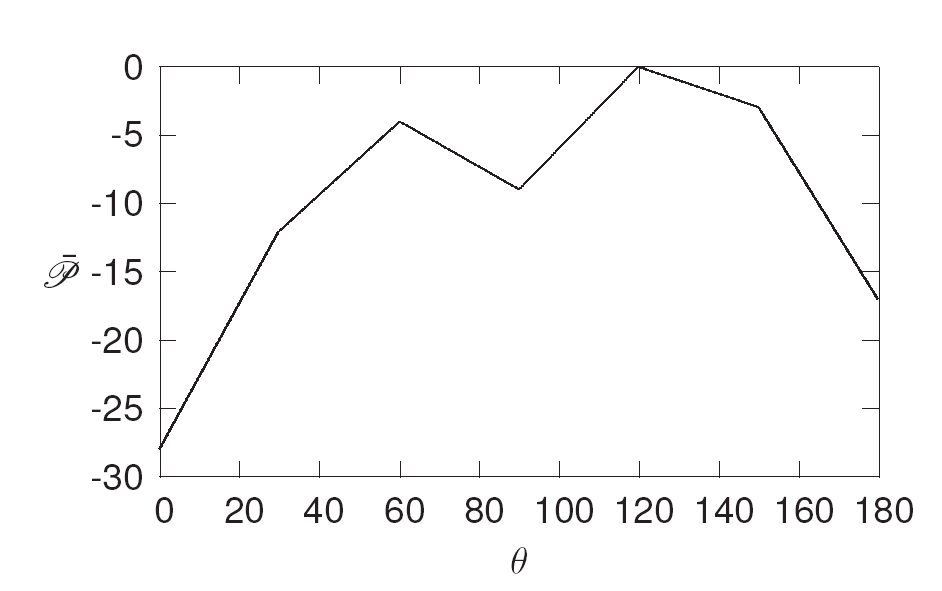Posted:4/08/2015 2:10PM

### The Creation of a 1 GHz Half-Wave Dipole Antenna

Mike Mclain discusses the creation of a 1 GHz half-wave dipole antenna and presents the necessary calculations associated with that antenna's development.

## Preface:

The purpose of this article is to discuss the development and creation of a 1 GHz half-wave dipole antenna from its conception to its physical hardware implementation. This article will accomplish this task by providing design calculations, experimental results, and diagrams of the materials utilized in the antennas physical implementation.

## General Design Calculations:

The first step needed to create a 1 GHz half-wave dipole antenna is to calculate the fundamental wavelength of the desired signal (in this particular case 1 GHz) by using the wavelength equation, as shown by:

$\lambda = \frac{v_p}{f}$

in which ($\lambda$) is the wavelength, ($v_p$) is the phase velocity, and ($f$) is the frequency.1 [p.15]

Likewise, the phase velocity ($v_p$) of the signal can then be calculated by using the phase velocity equation, as shown by:

$v_p = \frac{1}{\sqrt{\mu_r\mu_0\varepsilon_r\varepsilon_0}}$

in which ($\mu_r$) is the relative permeability, ($\mu_0$) is the permeability of free-space, ($\varepsilon_r$) is the relative permittivity, and ($\varepsilon_0$) is the permittivity of free-space.1 [p.15]

Conversely, substitution of these parameters into Equation: 2 results in the creation of:

$v_p = \frac{1}{\sqrt{\mu_r\mu_0\varepsilon_r\varepsilon_0}}\\\\ = \frac{1}{\sqrt{(1)(4\pi\times 10^{-7})(1)(8.854\times 10^{-12})}}\\\\ = 2.99796\times10^{8}$

, and substitution of these parameters into Equation: 1 results in the creation of:

$\lambda = \frac{v_p}{f}\\\\ = \frac{2.99796\times10^{8}}{1\times 10^{9}}\\\\ = .299796m$

Now, since most building materials are specified in inches rather than in metric values, conversion from meters to inches can be done by:

$1m \approx 39.3700787in$

and substitution of Equation: 5 into Equation: 4 results in the creation of:

$\lambda = .299796m\\\\ = \left(.299796m\right)\left(\frac{39.3700787in}{1m}\right)\\\\ = 11.803in$

.2

Likewise, (because a half-wave dipole antenna is being designed) the half-wavelength of the desired signal (1 GHz) can be calculated from Equation: 6, as shown by:

$\lambda_{\frac{1}{2}} = \frac{\lambda}{2}\\\\ = \frac{11.803in}{2}\\\\ = 5.90149in$

and similarly, the fundamental structure of a half-wave dipole antenna, as shown by:

, can be conceptually summarized as being an antenna with two conductive elements that are separated by a small gap (in the middle) to aid in defining the antenna's impedance. Furthermore, the half-wave dipole antenna gets its name from the fact that the total length of the antenna (including both radiating elements and the gap) is half the wavelength of the desired (in this case 1 GHz) transmitting/receiving frequency.

Conversely, because the total length of the antenna being created is half the wavelength of the desired 1 GHz signal, the half-wavelength value found in Equation: 7 was divided (once again) by one half in order to find the length of each dipole element as shown by:

$\lambda_{\frac{1}{4}} = \frac{\lambda_{\frac{1}{2}}}{2}\\\\ = \frac{5.90149}{2}\\\\ = 2.950745in$

. Likewise, because a finite gap must exist between the two antenna elements (and this gap is not accounted for within Equation: 8) some modifications to the length equation must be made to account for this gap. Conversely, since the gap shown (within Figure: 1) extends outwards from the center of the antenna symmetrically, the gap must also be divided by two in order to obtain the size (or half gap) that each antenna element needs to have subtracted from its element length in order to maintain the antenna's overall half-wavelength, as shown by:

$Gap_{\frac{1}{2}} = \frac{Gap}{2}$

.

Similarly, upon accounting for the half gap (observed within Equation: 9) in the element's quarter wavelength equation (defined within Equation: 8) results in the creation of the total element length equation, shown by:

$\lambda_{Gap} = \lambda_{\frac{1}{4}}-Gap_{\frac{1}{2}}\\\\ = 2.950745in-Gap_{\frac{1}{2}}$

## Performance Calculations:

. Now, because the gaps overall (or total) size will have real world design consequences, along with the fact that the mathematical equations needed to consider the size of the gap are very complicated (as observed upon examining :

$I_e(x^{'},y^{'},z^{'}) = \left\{\begin{array}{rc} \hat{a}_z\left\{I_0\sin\left[k\left(\frac{\ell}{2}-z^{'}\right)\right]+jpI_0\left[\cos\left(kz^{'}\right)-\cos\left(\frac{k}{2}\ell\right)\right]\right\} & 0\leq z^{'}\leq\frac{\ell}{2} \\ \hat{a}_z\left\{I_0\sin\left[k\left(\frac{\ell}{2}+z^{'}\right)\right]+jpI_0\left[\cos\left(kz^{'}\right)-\cos\left(\frac{k}{2}\ell\right)\right]\right\} & \frac{-\ell}{2}\leq z^{'}\leq 0 \end{array}\right.$

, which is the current distribution equation for a antenna with a gap where $p$ is an unspecified coefficient, likely empirical, that relates the gap to the antenna length), it is best to neglect the size of the gap when performing back of the envelop calculations and once the general design is known then a RF computational tool (like NEC or HFSS) can be utilized to analyze the effects the gap has on the overall design.3 [p.181]

Likewise, because of the overall mathematical complexity created by including the gap into the antenna's theoretical design, it is best (for the moment) to neglect the length of the gap within the fundamental design equations; however, it is also important to remember the existence of the gap and possible effects that the gap will have when fine tuning the design within software simulations.

Conversely, upon neglecting the gap from the design equations, the ideal current distribution (across the antenna) can be modeled mathematically, by:

$I_e(0,0,z^{'}) = \left\{ \begin{array}{rc}\hat{a}_z I_0\sin\left[k\left(\frac{\ell}{2}-z^{'}\right)\right] & 0\leq z^{'}\leq\frac{\ell}{2} \\ \hat{a}_zI_0\sin\left[k\left(\frac{\ell}{2}+z^{'}\right)\right] & \frac{-\ell}{2}\leq z^{'}\leq 0\end{array} \right.$

in which ($I_0$) is the current maximum, ($k$) is the wave number which is defined by:

$k = \frac{2\pi}{\lambda}$

, ($\ell$) is the length of the antenna, and ($z^{'}$) is a position on the antenna with respect to the z axis (or element axis).3 [pp.170,179]

Now, assuming a wavelength equal to the one calculated for 1 GHz (along with a unity maximum current) results in a current distribution plot which (when superimposed onto a figure of the dipole antenna) produces a graph as shown by:

, that conceptually illustrates the current distribution assumed for all mathematical calculations while designing the antenna.

Now that the current distribution has been assumed, the next thing that can be found is the magnetic vector potential which is described by:

$A_r = \frac{\mu_0I_0\cos(\theta)}{2\pi rk\sin^2(\theta)}e^{-jkr}\left[\cos\left(\frac{k\ell}{2}\cos(\theta)\right)-\cos\left(\frac{k\ell}{2}\right)\right]$

and :

$A_{\theta} = -\frac{\mu_0I_0}{2\pi rk}e^{-jkr}\left[\frac{\cos\left(\frac{k\ell}{2}\cos(\theta)\right)-\cos\left(\frac{k\ell}{2}\right)}{\sin(\theta)}\right]$

, in which ($r$) represents the distance away from the antenna.4

Likewise, graphing $|A_r|$ produces:Figure 3: Magnitude $|A_r|$ Plot of Magnetic Vector Potential.

, while graphing $|A_\theta|$ produces:Figure 4: Magnitude $|A_\theta |$ Plot of Magnetic Vector Potential.

; however, neither of these values are particularly useful (in their current context) because the magnetic vector potential is an intermediary step before obtaining values of electric and magnetic intensity.

Conversely, after performing a few mathematical operations that involve substitution with the magnetic vector potential equations, the electric intensity equation, as shown by:

$\bar{E} \approx j\eta\frac{I_0}{2\pi r}e^{-jkr}\left[\frac{\cos\left(\frac{k\ell}{2}\cos(\theta)\right)-\cos\left(\frac{k\ell}{2}\right)}{\sin(\theta)}\right]\hat{a}_{\theta}$

, and the magnetic intensity equation, as shown by:

$\bar{H} \approx \frac{jI_0}{2\pi r}e^{-jkr}\left[\frac{\cos\left(\frac{k\ell}{2}\cos(\theta)\right)-\cos\left(\frac{k\ell}{2}\right)}{\sin(\theta)}\right]\hat{a}_{\phi}$

, can be obtained, In which ($\eta$) is defined by:

$\eta = \sqrt{\frac{\mu_r\mu_0}{\varepsilon_r\varepsilon_0}}$

.4 Likewise, Graphing $|\bar{H}|$ produces:Figure 5: Magnitude $|\bar{H}|$ Plot of Magnetic Intensity.

, while graphing $|\bar{E}|$ produces:Figure 6: Magnitude $|\bar{E}|$ Plot of Electric Intensity.

, noting that both graphs have a similar shape (but $\bar{H}$ varies with $\hat{a}_{\phi}$ while $\bar{E}$ varies with $\hat{a}_{\theta}$) and such similarities should be expected given the direct connection between the magnetic and electric fields.

Similarly, with values of magnetic and electric intensity known, the time-average power density can be calculated by:

$\mathscr{\bar{P}}_{av} = \frac{\eta\left|I_0\right|^2}{8\pi^2r^2}\left[\frac{\cos\left(\frac{k\ell}{2}\cos(\theta)\right)-\cos\left(\frac{k\ell}{2}\right)}{\sin(\theta)}\right]^2\hat{a}_r$

.4

Likewise, graphing $|\mathscr{\bar{P}}_{av}|$ produces:Figure 7: Magnitude $|\mathscr{\bar{P}}_{av}|$ Plot of Time-Average Power Density.

, and the time-average power density can now be used to calculate the radiation intensity, as shown by:

$\bar{U} = r^2|\mathscr{\bar{P}}_{av}|\\\\ = \frac{\eta\left|I_0\right|^2}{8\pi^2}\left[\frac{\cos\left(\frac{k\ell}{2}\cos(\theta)\right)-\cos\left(\frac{k\ell}{2}\right)}{sin(\theta)}\right]^2$

.4

Conversely, graphing $|\bar{U}|$ produces:Figure 8: Magnitude $|\bar{U}|$ Plot of Radiation Intensity.

which, because of the unity value selected for ($r$), the time-average power density is identical to the radiation intensity. Likewise, more mathematics can be applied to the time-average power density in order to obtain the total radiation power, as shown by:

$\mathscr{\bar{P}}_{rad} = \frac{\eta\left|I_0\right|^2}{4\pi}\left\{C+\ln(k\ell)+\frac{1}{2}\sin(k\ell)\left[S_i\left(2k\ell\right)-2S_i\left(k\ell\right)\right]\right. \\ +\left.\frac{1}{2}\cos\left(k\ell\right)\left[C+\ln\left(\frac{k\ell}{2}\right)+C_i\left(2k\ell\right)-2C_i\left(k\ell\right)\right]-C_i(k\ell)\right\}$

, in which ($C_i(x)$) is defined by:

$C_i(x) = \int_x^{\infty}\frac{\cos(y)}{y}dy$

, ($S_i(x)$) is defined by:

$S_i(x) = \int_0^x\frac{\sin(y)}{y}dy$

, and ($C$) is defined by:

$C = 0.5772$

.4

Similarly, substitution into Equation: 21 results in the creation of:

$\mathscr{\bar{P}}_{rad} = 36.5393$

, and now that the value for radiation intensity and total radiated power has been found, directivity can be calculated by:

$D = \frac{4\pi\bar{U}}{\mathscr{\bar{P}}_{rad}}$

.4 Likewise, Graphing $|D|$ produces:Figure 9: Magnitude $|D|$ Plot of Directivity.

, which is the same plot observed within Figure: 8 but this plot is scaled by a numerical factor. Conversely, (at this point) the radiation resistance can be calculated by:

$R_r = \frac{2\mathscr{\bar{P}}_{rad}}{|I_0|^2}$

, and further substitution results in obtaining the value of:

$R_r \approx 73.0785$

.4

Now, assuming a half-wavelength current density (as defined within Equation: 12 the input resistance for the antenna can be found by application of:

$R_{in} = \frac{|I_0|^2R_r}{|I_0|^2\sin^2\left(\frac{k\ell}{2}\right)}$

and further substitution results in obtaining the value of:

$R_{in} \approx 73.0785$

.4

## Experimental Results:

After all of the mathematical calculations were simulations can be performed in both HFSS and NEC. The first set of simulations i performed were done in NEC, as shown by:

, in which the values needed to create a half-wave dipole antenna (from the math above) were entered into the NEC software. Likewise, upon running a NEC simulation, a graph of both the two dimensional total gain plot, as shown by:

, and a three dimensional total gain plot, as shown by

were obtained.

Similarly, a second set of simulations were performed in HFSS and a dipole structure was created, as shown by:

, that was separated by a small gap, as shown by:

and simulations were performed on the model.

Likewise, a three dimensional total gain plot for the HFSS simulation was found, as shown by:

, which (upon comparison with Figure: 12) appears to match the overall shape of the NEC 3D simulation. While HFSS is a more rigorous simulation software than NEC; however, one issue that arose (upon comparing results between the two) was a discrepancy between the input impedance calculated by HFSS and NEC, although the mathematics (provided above) seemed to agree more with the NEC simulation (vs. the HFSS simulation) yet HFSS is designed to account for more complex EM effects and this fact must also be considered while evaluating results.

Note: during this part of the design process, I considered utilizing a single stub tuner to match the impedance of the antenna to a 50$\Omega$ coaxial cable; however, the occurrence of such discrepancies (between the two simulations) made such notions a very bad idea, especially since a exact input impedance is required for single stub tuning to work correctly. Likewise, because the only option available (to obtain a exact input impedance given such discrepancies) was to physically build the antenna and measure the input impedance (prior to stubing), thus such options were not very viable (because of time and material constraints) so i decided not to utilize a single stub tuner for this particular antenna design.

## Hardware Implementation:

Conversely, one of the first hardware challenges that needed to be resolved (when building the antenna) was deciding upon the type of coaxial cable that was going to be used since both $50\Omega$ and $75\Omega$ coaxial cable was available for this particular antenna design.

Likewise, originally $50\Omega$ coaxial cable was selected (in conjunction with a single stub tuner in order to match the antenna impedance to the coaxial cable impedance); however, (as discussed earlier) problems with knowing the physical impedance of the antenna made this selection a poor choice overall and the $50\Omega$ coaxial cable was then changed to $75\Omega$ coaxial cable at the cost of having a higher reflection coefficient between the RF 1 GHz power supply (that had a $50\Omega$ input impedance) and the $75\Omega$ coaxial cable.

Similarly, after deciding upon the $75\Omega$ coaxial cable, additional information about the dielectric of the cable needed to be found and some modifications made to the phase velocity calculation and to the wavelength calculation in order for a RF balun to be created correctly using the selected $75\Omega$ coaxial cable, as shown by:

$\varepsilon_{r_{Polyethylene}} = 2.25$

,:

$v_p = \frac{1}{\sqrt{\mu_r\mu_0\varepsilon_r\varepsilon_0}}\\\\ = \frac{1}{\sqrt{(1)(4\pi\times 10^{-7})(2.25)(8.854\times 10^{-12})}}\\\\ = 1.9986\times10^8$

,:

$\lambda = \frac{v_p}{f}\\\\ = \frac{1.9986\times10^{8}}{1\times 10^{9}}\\\\ = .1999m\\\\ = \left(.1999m\right)\left(\frac{39.3700787in}{1m}\right)\\\\ = 7.8687in$

, and by:

$\lambda_{\frac{1}{2}} = \frac{\lambda}{2}\\\\ = \frac{7.8687in}{2}\\\\ = 3.9343in$

Likewise, because the half-wave dipole must be driven (by the 1 GHz signal) differentially, i decided that a half-wavelength coaxial balun would be utilized, as shown by:

, because constructing this type of balun is relatively simplistic (when compared to the bazooka balun) and is easier to understand at a conceptual level.3 [p.541]

Conversely, upon building the half-wavelength balun and assembling the half-wave dipole (using the numbers calculated from above) resulted in the creation of:

, in which the balun assembly (within the photo) is observed to be a looped coaxial wire (noting that the electrical attachment from the balun to the antenna assembly was done experimentally via a twisted pair (at $\approx 70\Omega$ impedance) transmission structure to ensure that only a TEM mode of RF operation was in effect.

Likewise, the overall antenna was mounted on a PVC pipe stand to allow for easy deployment in an outside test environment, as shown by:Figure 18: Overall Body Shot of the designed Half-Wave Dipole Antenna.

.

## Experimental Measurements:

Conversely, this antenna was tested in a open outdoor area (to avoid RF reflections) at 1 GHz and a 3D radiation pattern was observed from a series of time average power density measurements obtained via a RF signal strength analyzer.

Likewise, in order to obtain this information, the antenna was first mounted with the end-fire elements pointed along the z axis (towards the sky and ground) and a RF signal strength analyzer was moved along a fixed radius (outside the transmission near field) while the time average power density (in dB) was recorded at fixed intervals, as shown by:Figure 19: Measured $\mathscr{\bar{P}}_{av}$ Plot of Time-Average Power Density in dB Over $\phi$ Axis.

. Similarly, after the $\phi$ axis time-average power density measurements were taken, the antenna was remounted with the end-fire elements pointed along the y/x axis (towards the horizon) and a RF signal strength analyzer was moved along a fixed radius (outside the transmission near field) while the time average power density (in dB) was recorded at fixed intervals, as shown by:Figure 20: Measured $\mathscr{\bar{P}}_{av}$ Plot of Time-Average Power Density in dB Over $\theta$ Axis.

.

## Conclusion:

Based upon the measurements obtained within Figure: 19 it becomes apparent that the created dipole antenna is functioning in a manner similar to the HFSS/NEC predicted results (an attribute that should be expected) given the consistent time average power observed across the $\phi$ axis (noting that the minor 5 dB deviations observed are likely the combined result of hardware defects, mathematical approximations, impedance imbalances, and testing apparatus errors. Likewise, the $\theta$ axis measurements (obtained within Figure: 20) are also consistent with the HFSS/NEC predicted results (as dipole antennas do not transmit from an end-fire configuration, thus the transmission power falls off rapidly at the end of each antenna element).

## Acknowledgments

Special thanks to my friend Aaron Hatley for his help in the development, design, construction, and testing of this antenna!

## References:

 D. M. Pozar, Microwave Engineering. John Wiley & Sons, Inc, third ed., 2005